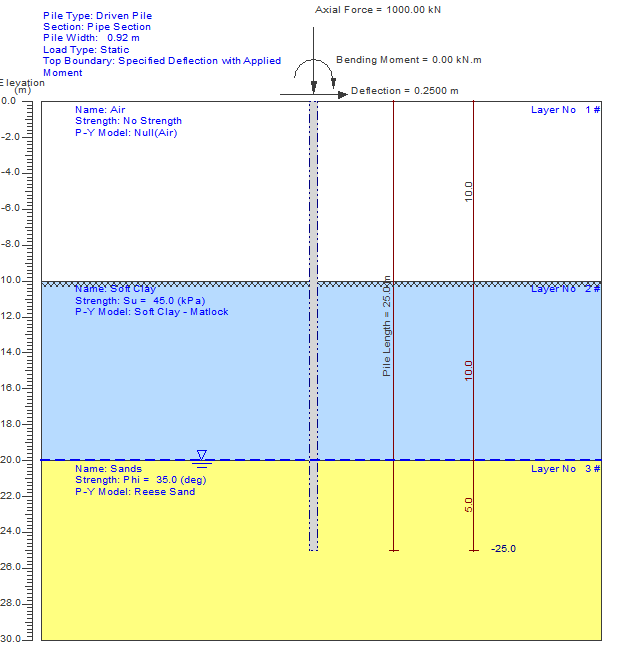top of page

The lateral displacement of pile foundation often shall be checked in the routine foundation design and the geotechnical engineers need to ensure that the relevant displacement compliance criterion can be satisfied. It is a general practice to ignore the P-Delta effect in the pile design with performing first-order analysis as the displacement criterion of limiting the pile deflection to within the range of 20 to 25 mm acts as a means to control the P-delta effect.However, it would be very useful if the analysis of P-Delta effect can be readily carried out in the design process where the piles can be designed according to the analysis results. As a result, the limiting criterion on the allowable displacement can be relaxed or waived entirely if the structural capacity is found adequate.

PileLAT program includes the P-Delta effect in the analysis of bending moment for laterally loaded piles under axial loading. The governing differential equation for the laterally loaded pile under axial loading is shown as below, in which P is the constant axial loading on the pile.This equation is solved numerically with using finite element method through iterative procedures in order to consider the nonlinear response of soils modeled by P-Y curves in the PileLAT program.

This technical blog presents an example to demonstrate the capability of PileLAT for considering P-Delta effect for the piles with both lateral and axial loading. The pile geometry and soil profile for the analysis problem are shown in the figure as below. The pile is an elastic steel pipe section, 910 mm diameter with a wall thickness of 12.5 mm. The pile length is 20 m and the pile extends 10 m above the ground surface. The upper soil layer is soft clay and the lower soil layer is sand.In this problem, the axial load applied at the pile top is 1000 kN. A specified horizontal displacement of 250 mm with zero bending moment are applied to the pile top.The calculated shear force at the pile top, Vtop, is 136.772 kN and the lateral displacement at the ground surface level is 41.048 mm. Therefore, the bending moment at the ground surface level due to the shear force at the pile top can be calculated as: Mv = Vtop x Le = 136.772 x 10.0 = 1367.72 kN.m.

The additional bending moment from P-Delta effect due to the axial loading can be estimated as the relative displacement between the pile top and ground surface level multiplied by the axial loading: Mp = (0.249999 - 0.041048) x 1000 = 208.951 kN.m The total bending moment at the ground surface level (10 m below the pile top) is therefore calculated as: M = Mv + Mp = 1367.72 + 208.951 = 1576.7 kN.m The bending moment computed by PileLAT directly is 1576.3 kN.m at the ground surface level, which is almost the same.

The figure below shows the detailed outputs from the analysis for laterally loaded piles under axial loading with considering P-Delta effect.1,947 views